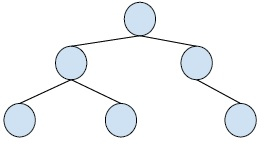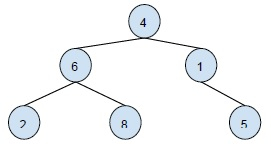# Print cousins of a given node in Binary Treein C++

Binary Tree is a special tree whose every node has at max two child nodes. So, every node is either a leaf node or has one or two child nodes.

Example,In this problem, we are given a binary tree and we have a node of the tree and we have to find the cousin nodes of the node. No sibling nodes are to be printed for the binary tree.

Let’s take an example,For the above binary tree, the cousin node is 5.

To make the concept more clear let’s describe cousin node. In a binary tree, two nodes are said to be cousin nodes if they are at the same level (depth) in the binary tree but don't have the same parent node.

Now, let’s create a solution to this problem.

Here we have to print all the nodes at the same level of the node i.e. all the nodes that are at the same distance from the root node. But we have to eliminate the node that has the same parent as the node itself. For this, we will first find the level of the node and then print all the nodes except the node itself and the node with the same parent.

## Example

Now, let's create a program based on this logic −

#include <bits/stdc++.h>
using namespace std;
struct Node{
int data;
Node *left, *right;
};
Node *newNode(int item){
Node *temp = new Node;
temp->data = item;
temp->left = temp->right = NULL;
return temp;
}
int levelOfNode(Node *root, Node *node, int level){
if (root == NULL)
return 0;
if (root == node)
return level;
int downlevel = levelOfNode(root->left,
node, level + 1);
if (downlevel != 0)
return downlevel;
return levelOfNode(root->right, node, level + 1);
}
void printCousin(Node* root, Node *node, int level){
if (root == NULL || level < 2)
return;
if (level == 2){
if (root->left == node || root->right == node)
return;
if (root->left)
cout << root->left->data << " ";
if (root->right)
cout << root->right->data;
}
else if (level > 2){
printCousin(root->left, node, level - 1);
printCousin(root->right, node, level - 1);
}
}
void cousinNode(Node *root, Node *node){
int level = levelOfNode(root, node, 1);
printCousin(root, node, level);
}
int main(){
Node *root = newNode(11);
root->left = newNode(15);
root->right = newNode(4);
root->left->left = newNode(3);
root->left->right = newNode(7);
root->left->right->right = newNode(9);
root->right->left = newNode(17);
root->right->right = newNode(8);
root->right->left->right = newNode(5);
cout<<”The cousin nodes are : \t”
cousinNode(root, root->right->right);
return 0;
}

## Output

The cousin nodes are : 3 7